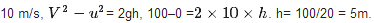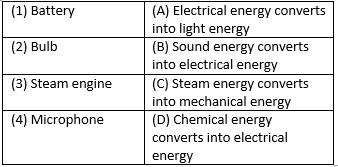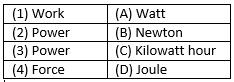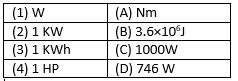Courses

# HOTS Questions: Work & Energy

## 25 Questions MCQ Test General Science(Prelims) by IRS Divey Sethi | HOTS Questions: Work & Energy

Description
This mock test of HOTS Questions: Work & Energy for UPSC helps you for every UPSC entrance exam. This contains 25 Multiple Choice Questions for UPSC HOTS Questions: Work & Energy (mcq) to study with solutions a complete question bank. The solved questions answers in this HOTS Questions: Work & Energy quiz give you a good mix of easy questions and tough questions. UPSC students definitely take this HOTS Questions: Work & Energy exercise for a better result in the exam. You can find other HOTS Questions: Work & Energy extra questions, long questions & short questions for UPSC on EduRev as well by searching above.
QUESTION: 1

### Work done by centripetal force is

Solution:

When a body moves on a circular path. The force that acts on the revolving body towards the center is called centripetal force. As the force acts as right angle to the direction of motion, so work done is 0.

QUESTION: 2

### Statement A: Any physical or mental activity is termed as work done in physics. Statement B: Work is said to be + ve work done when the body is displaced in the direction of applied force. Which of the two statements is true?

Solution:

In physics, a work is said to be done when there is some displacement on the body on which force is applied. Work done is +ve when the body is displaced in direction of applied force.

QUESTION: 3

### P. E of your body is maximum when you

Solution:

Potential Energy of a body = mgh, hence the potential energy is maximum when you are standing, given that acceleration due to gravity and mass remain constant.

QUESTION: 4

According to the law of conservation of energy

Solution:

According to the law of conservation of energy, energy neither be created nor be destroyed, it can be transformed from one form to another. So, all the statements are true.

QUESTION: 5

For what value of .ϕ. is work done maximum?

Solution:

Work done when a body moves at an angle to the direction of force is W=Fcosϕ×s. The value of Cos is maximum for 00. So, work done will be maximum for this angel.

QUESTION: 6

Find the correct statement

Solution:

One Giga watt is equal to 109 watt or 106 kilowatt. The rate of doing work is called as power. Hence both statements are correct.

QUESTION: 7

Statement A: An aeroplane flying at an altitude possesses only K. E.
Statement B: An aeroplane flying at an altitude possesses both K. E and P. E.
Which of the two statement is true

Solution:

An aeroplane flying at an altitude possesses both kinetic energy and potential energy. Kinetic energy is due to mass and velocity and potential energy is due to mass and height.

QUESTION: 8

A ball is dropped from a height ‘h’. The velocity of the ball when it reaches the ground is 10 m/s. Find ‘h’ (g = 10ms−2)

Solution:

Initial velocity (u)= 0, final velocity (v) =QUESTION: 9

1k w h = ?

Solution:

1 kwH = 1000 W×60×60 s = 3600000J = 3.6 × 106  J. one watt is equal to one joule per second.

QUESTION: 10

An electric lamp of 100w is used for 5 hours per day calculate the units of energy consumed by lamp in one day

Solution:

Power of lamp = 100w = 100/1000 = 0.1Kw, time= 5 hrs. Electrical energy = power×timepower×time, E = 0.1kw×5h = 0.5 kw–h. = 0.5 unit.

QUESTION: 11

Find the incorrect statement

Solution:

The potential energy of a freely falling object decreases progressively due to decrease in height. Kinetic energy is converted into heat and sound energy when a freely falling object on reaching the ground. Work is done only when change in position occurs.

QUESTION: 12

Statement A: Mechanical energy of a system is always conserved.
Statement B: The total energy of the system is always conserved.
Which of the two statements is true?

Solution:

The total energy of the system is always conserved. It is just transformed from one form to other form. Mechanical energy of a system is not conserved. It is converted into other form of energy.

QUESTION: 13

1 W. S = ________?

Solution:

1 watt second (W.S) = 1J/s×s= 1 joule.

QUESTION: 14

In which of the following activities the work is said to be done?

Solution:

No work is done in reading a book, pulling wall of house and walking on a level road with book on his head as there is no change in the position of object on which force is applied. Stretching string causes displacement, so it is a work.

QUESTION: 15

When you compress a coil spring you do work on it. The elastic P. E energy

Solution:

When you compress a coil spring you do work on it. The elastic potential energy increases.

QUESTION: 16

Match the following with correct response.Solution:
QUESTION: 17

A certain household has consumed 250 units of energy during a month. How much energy is this in joules?

Solution:

1 unit (Kw-H) = 3.6×106 joule. Therefore, 250 units = 250×3.6×106joule
= 9×108 Joule.

QUESTION: 18

Match the following with correct response.Solution:
QUESTION: 19

P.E of a spring increases when it is in the state of

Solution:

Potential energy of a spring increases when it is in the state of expansion and compression. Elastic potential energy increases with compression and gravitational potential energy increases on expansion.

QUESTION: 20

Work done by the gravitational force of earth on a satellite along a circular path is

Solution:

The gravitational force of the earth on a satellite along a circular path is O as gravitational force of earth acts on right angle which is O for cos.

QUESTION: 21

9800 joule of energy was spent to raise a mass of 50kg, the mass was raised to a height of

Solution:

Potential energy = mgh, here, RE = 9800 j. mass = 50kg, g = 9.8m/s2. Therefore, 9800 = 50 x 9.8 x h, h= 9800/50 x 9.8 = 20m.

QUESTION: 22

Match the following with correct response.Solution:
QUESTION: 23

What happens to the kinetic energy of a freely falling object which eventually stops on reaching the ground?

Solution:

The kinetic energy of a freely falling object which eventually stops on reaching the ground changes into heat and sound energy.

QUESTION: 24

The linear momentum of a body of mass 2kg is 16kgms−1. What is its K. E?

Solution:

Momentum = mass x velocity, velocity = momentum/ mass = 16/2 = 8m/s. Kinetic energy = ½ mv2. K.E = ½ x2 x 82 = 64 J.

QUESTION: 25

Which of the following is unit of energy
A. Newton metre
B. Joule
C. Kilowatt hours
D. Kilowatt

Solution:

We know that, joule, newton metre and kilowatt hour are the units of energy and the kilowatt is the unit of power.